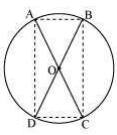# AC and BD are chords of a circle which bisect each other. Prove that (i) AC and BD are diameters; (ii) ABCD is a rectangle.Given: Two chords AC and BD bisects each other i.e OA=OC,OB=OD

To prove: In ∆AOB and COB

AO=CO ——-[given]

∠AOB=∠COD ——[vertically opposite angle]

OB=OD ——–[given]

∴∆AOB≅∆COB ——[s.s.s]

AB=CD ——[C.P.C.T.] ————–(i)

similarly ∆AOD≅∆COB (S.A.S)

From (i) and (ii)

ABCD is a ||gm

We know that ABCD is cyclic quadrilateral

∴∠A+∠C=180°

∠B+∠B=180°

2∠B=180°

∠B=180°/2

∠B=90°

∴ ∠A and ∠B lies in a semicircle

→ AC and BD are diameter of circle.

ii) Since ABCD is a ||gm and ∠A=90°

∴ ABCD is a rectangle.